# CAT 2017 – Slot 1 – Quantitative Ability – Suppose, log(base3)x = log(base12)y = a

Q. 1: Suppose, log(base3)x = log(base12)y = a, where x, y are positive numbers. If G is the geometric mean of x and y, and log(base6)G is equal to
A) √a
B) 2a
C) a/2
D) a

x=3^a and y=12^a

G = √(3^a * 12^a) = 6^a

Log (base 6) 6^a = a

Option (D)

##### Q. 2: If x + 1 = x^2 and x > 0, then 2x^4 isA) 6 + 4√5B) 3 + 5√5C) 5 + 3√5D) 7 + 3√5

x+1=x^2

Find out the roots of x = [1+/- root(5)]/2

X2 = [3 +/- √5]/2

X4 = [7 +/-3√5]/2

2×4 = 7 +/- 3√5

As the only option is 7 + 3√5 So, we go with that.

Option (D)

Q. 3: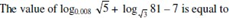A) 1/3
B) 2/3
C) 5/6
D) 7/6

Explanation: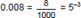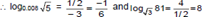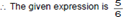Q. 4: If 9^(2x – 1) – 81^(x-1) = 1944, then x is
A) 3
B) 9/4
C) 4/9
D) 1/3

9^(2x-1) – 9^(2x-2) = 1944

It can be written as 3^(4x)/9 – 3^(4x)/81 = 1944

8(3^(4x)/81) = 1944

x =9/4

Option (B)

Q. 5: The number of solutions (x, y, z) to the equation x – y – z = 25, where x, y, and z are positive integers such that x ≤ 40, y ≤ 12, and z ≤ 12 is
A) 101
B) 99
C) 87
D) 105

x = 25 + y + z. The possible values of x, y, z and the corresponding number of values of y, z are tabulated below (x, y, z are positive integers).

We see that 27 ≤ x ≤ 40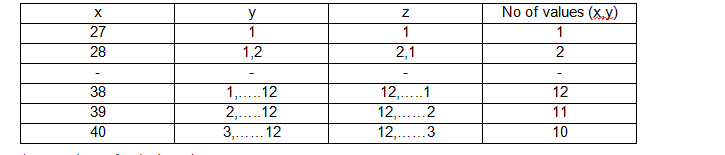The number of solutions is 1 + 2 + …… + 12 + 11 + 10 = 78 + 21 = 99

Option (B)

##### Checkout Other Questions of CAT 2017 Slot 1 Paper:

Verbal Ability :              |   Q.01- Q.06  |  Q.07- Q.12  |  Q.13- Q.18  |  Q.19- Q.21  |  Q.22- Q.24  |  Q.25- Q.29  |  Q.30 – Q.34  |

Logical Reasoning :    |   Q.29 – Q.32  |

Quantitative Aptitude: |

### Free Material Area